Courses

# RD Sharma Solutions - 17.5, Constructions, Class 7, Math Class 7 Notes | EduRev

## RD Sharma Solutions for Class 7 Mathematics

Created by: Abhishek Kapoor

## Class 7 : RD Sharma Solutions - 17.5, Constructions, Class 7, Math Class 7 Notes | EduRev

The document RD Sharma Solutions - 17.5, Constructions, Class 7, Math Class 7 Notes | EduRev is a part of the Class 7 Course RD Sharma Solutions for Class 7 Mathematics.
All you need of Class 7 at this link: Class 7

#### Question 1:

Draw a right triangle with hypotenuse of length 5 cm and one side of length 4 cm.

Steps of construction:

1. Draw a line segment QR = 4 cm.
2. Draw ∠QRX of measure 90°°.
3. With centre Q and radius PQ = 5 cm, draw an arc of the circle to intersect ray RX at P.
4. Join PQ to obtain the desired triangle PQR.
5. PQR is the required triangle.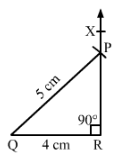#### Question 2:

Draw a right triangle whose hypotenuse is of length 4 cm and one side is of length 2.5 cm.

Steps of construction:

1. Draw a line segment QR = 2.5 cm.
2. Draw ∠QRX of measure 90°°.
3. With centre Q and radius PQ = 4 cm, draw an arc of the circle to intersect ray RX at P.
4. Join PQ to obtain the desired triangle PQR.
5. PQR is the required triangle.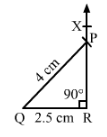#### Question 3:

Draw a right triangle having hypotenuse of length 5.4 cm, and one of the acute angles of measure 30°.

Let ABC be the right triangle at A such that hypotenuse BC = 5.4 cm. Let ∠C = 30°
Therefore∠A+∠B+∠C =180°
∠B =180−30−90 = 60°
Steps of construction:

1. Draw a line segment BC = 5.4 cm.
2. Draw angle CBY = 60o.
3. Draw angle BCX of measure 30o with X on the same side of BC as Y.
4. Let BY and CX intersect at A.

Then ABC is the required triangle.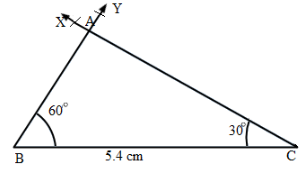#### Question 4:

Construct a right triangle ABC in which AB = 5.8 cm, BC = 4.5 cm and ∠C = 90°.

Steps of construction:

1. Draw a line segment BC = 4.5 cm.
2. Draw ∠BCX of measure 90°°.
3. With centre B and radius AB =5.8 cm, draw an arc of the circle to intersect ray BX at A.
4. Join AB to obtain the desired triangle ABC.
5. ABC is the required triangle.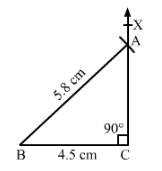#### Question 5:

Construct a right triangle, right angled at C in which AB = 5.2 cm and BC = 4.6 cm.

Steps of construction:

1. Draw a line segment BC = 4.6 cm.
2. Draw ∠BCX of measure 90°°.
3. With centre B and radius AB = 5.2 cm, draw an arc of the circle to intersect ray CX at A.
4. Join AB to obtain the desired triangle ABC.
5. ABC is the required triangle.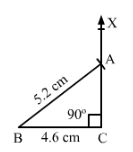97 docs

,

,

,

,

,

,

,

,

,

,

,

,

,

,

,

,

,

,

,

,

,

,

,

,

,

,

,

,

,

,

;